# Translation of programs

(diff) ← Older revision | Latest revision (diff) | Newer revision → (diff)

The translation of programs in programming, also called compilation of programs, is a systematic process that transforms any program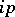in the input algorithmic languageinto some program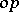in the object language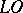; furthermore, both programsandshould realize the same function, that is, ifis the input data of the program, then.

A translation of programs in the theory of computable functions and theory of algorithms (cf. Algorithms, theory of; Computable function) is any mapping of one enumeration of computable functions to another that preserves the property that the image and pre-image are the numbers of the same function (the presence of an effective translating mapping is also called reducibility of one enumeration to another).

In programming practice, the programming language used by the human being is usually the input language, while the object language is usually the language that is carried out directly by the machine programs. The translation of programs itself is, as a rule, carried out automatically, that is, by means of a programin some realization language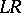, called the translator (or compiler), that is,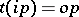. The systematic development of translators for any input language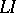from some class of languages is what constitutes automatic programming, and the corresponding devices of such a development are called systems of compiler construction, or compilers of compilers,:. Here, the realization language either contains the object language or coincides with it: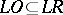.

The notion of a translation of programs (reducibility) in the theory of computable functions leads to the notion of principal enumerations, that is, enumerations to which any other enumeration of some class can be reduced. The existence has been proved of principal computable enumerations for all concrete models of computable functions; in particular for partial recursive functions and for Turing machines. In turn, the existence of principal computable enumerations is enabled by the ability of computable functions to perform so-called partial computations, that is, by the existence of a general recursive function (in programming, a partial computer; in the theory of computable functions, an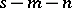-function)such that if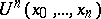is a universal function for computable functions ofvariables, then for any computable functionof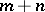variables and with number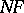, one has the identity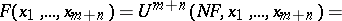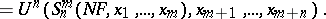As is obvious from this identity, a partial computer constructs from a program ofvariables and given values ofvariables a program of a function ofvariables obtained from the original program by associatingof its arguments with these values. The result of a partial computation is called the projection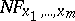of the programon the given values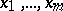ofof its arguments. The existence of principal computable enumerations (see , Chapt. 1, Sect. 2) and partial computers (see , Sect. 65), as well as their connection (see , Sect. 11, Theorem 3) is one of the fundamental aspects of the theory of computable functions.

There is a direct relationship between problems of practical translation in programming and partial computations (see ). Suppose that the realization languagehas a principal computable enumeration and let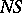be the program of a partial computer forexpressed in the same language. Suppose further that the input languageis defined by a program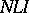of its universal function expressed in an object subsetof, that is,. (In programming, such a program is called an interpreter of the input language.) Then the following relations hold: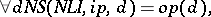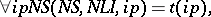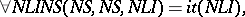that is, the object program is the projection of the interpreter of the input language onto the input program; the compiler is the projection of the partial computer onto the interpreter of the input language; while the compiler of compilers is the projection of the partial computer onto itself.

How to Cite This Entry:
Translation of programs. Encyclopedia of Mathematics. URL: http://encyclopediaofmath.org/index.php?title=Translation_of_programs&oldid=12660
This article was adapted from an original article by A.P. Ershov (originator), which appeared in Encyclopedia of Mathematics - ISBN 1402006098. See original article